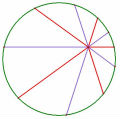# 101南港高工

## 101南港高工

101南港高工教甄數學試題、解答

【註：weiye 將原始檔案壓縮處理，減少檔案大小後，已上傳於附件。2012.06.26 15:50】

#### 附件101南港高工.pdf (636.84 KB)

2012-6-26 15:48, 下載次數: 7775

TOP

## 問題請教

4 ,11,12,13,16(完全不知從何下手)

...

TOP

## 回復 2# chiang 的帖子

奇奇偶，奇奇偶，奇奇偶，奇奇偶，奇奇偶，
按此規則即可推出答案

TOP

## 回復 2# chiang 的帖子

TOP

##### 引用:

4 ,11,12,13,16(完全不知從何下手)

...

#16

TOP

##### 引用:

#12

k1=5+x1 ,k2=5+x2 ,k3=5+x3
k4=5-x1 , k5=5-x2 , k6=5-x3

=(5+x1)+(5+x2)+(5+x3)+(5-x1)+(5-x2)+(5-x3)
=5*6
=30

TOP

 jmfeng2001發私訊 加為好友 目前離線 7# 大 中 小 發表於 2012-6-27 13:53  只看該作者 第11題...還不熟悉語法...希望你看的懂 設交點為A(1+a,1+b),B(1-a,1-b) 代入雙曲線得a=4b再代入得b^2=7/12 AB=根號((2a)^2+(2b)^2)     =根號17b^2     =根號119/3 另外,想請問各位老師...第6,7,8題 UID905 帖子22 閱讀權限10 上線時間98 小時 註冊時間2011-7-13 最後登入2014-8-14  查看詳細資料 TOP
 andyhsiao發私訊 加為好友 目前離線 8# 大 中 小 發表於 2012-6-27 18:11  只看該作者 第6題..參考看看...有錯請訂正^^13.jpg (53.97 KB) 2012-6-27 18:11 UID790 帖子49 閱讀權限10 上線時間70 小時 註冊時間2011-5-17 最後登入2012-7-27  查看詳細資料 TOP
 andyhsiao發私訊 加為好友 目前離線 9# 大 中 小 發表於 2012-6-27 18:33  只看該作者 第16題...參考101的P109頁...演練題第四題 UID790 帖子49 閱讀權限10 上線時間70 小時 註冊時間2011-5-17 最後登入2012-7-27  查看詳細資料 TOP
 老王 老王發私訊 加為好友 目前離線 10# 大 中 小 發表於 2012-6-27 18:42  只看該作者 第24題 $$\displaystyle \Delta BCH:\Delta ACH:\Delta ABH=\tan A:\tan B:\tan C=2:3:1$$ 設 $$\displaystyle \tan A=2k, \tan B=3k, \tan C=k$$ 又 $$\displaystyle A+B+C=\pi$$ 所以 $$\displaystyle \tan A+\tan B+\tan C=(\tan A)(\tan B)(\tan C)$$ $$\displaystyle 6k=6k^3$$ $$\displaystyle k=1$$ $$\displaystyle \tan A=2, \tan B=3, \tan C=1$$ $$\displaystyle \sin A=\frac{2}{\sqrt5}, \sin B=\frac{3}{\sqrt{10}}, \sin C=\frac{1}{\sqrt2}$$ $$\displaystyle a:b:c=2\sqrt2:3:\sqrt5$$ [ 本帖最後由 老王 於 2012-6-27 06:44 PM 編輯 ] 名豈文章著官應老病休飄飄何所似Essential isolated singularity UID261 帖子308 閱讀權限10 上線時間943 小時 註冊時間2009-5-14 最後登入2014-3-17  查看詳細資料 TOP
﻿